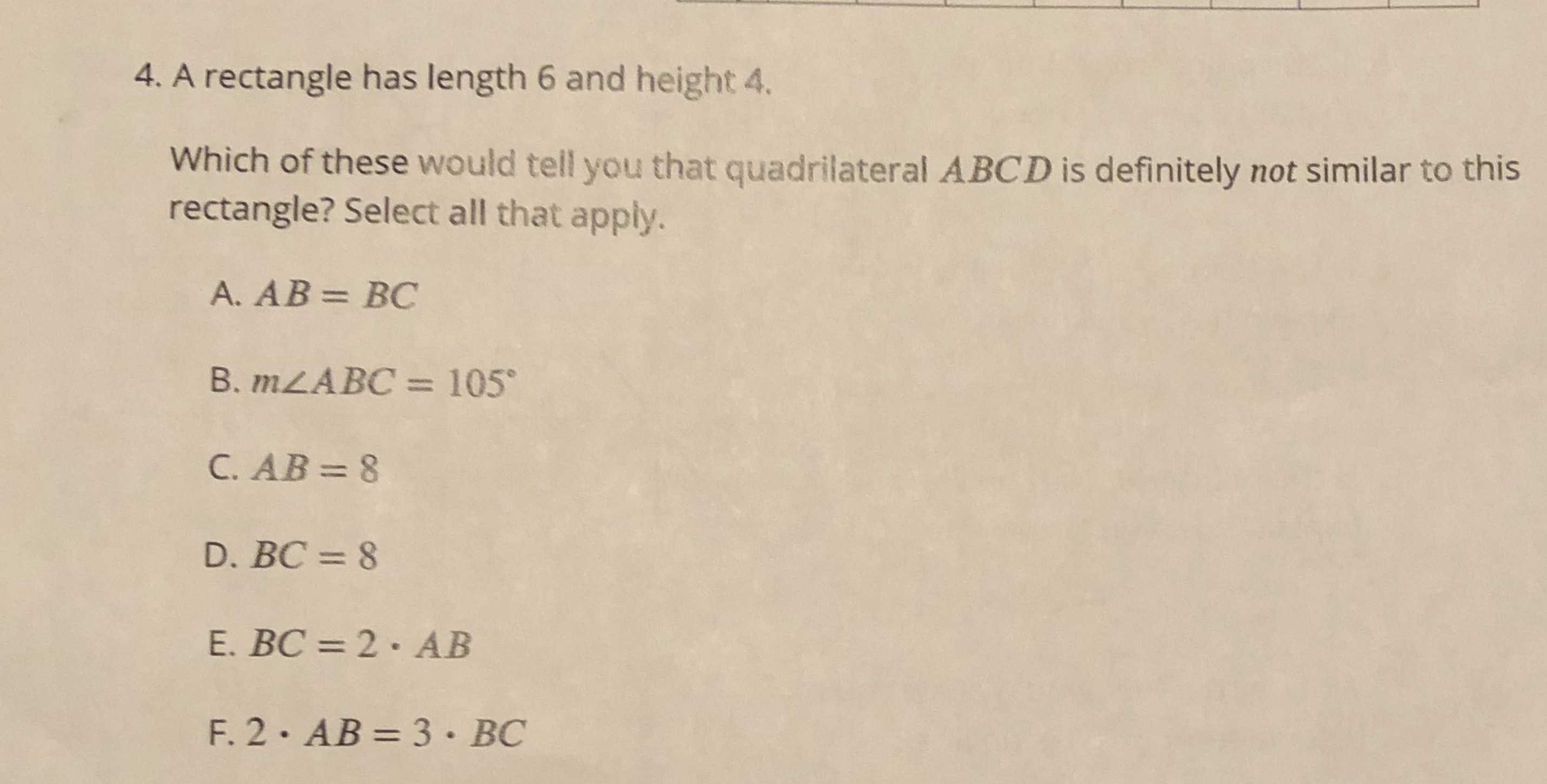### Still have math questions?

Trigonometry
QuestionA rectangle has length $$6$$ and height $$4 .$$

Which of these would tell you that quadrilateral $$A B C D$$ is definitely not similar to this rectangle? Select all that apply.

A. $$A B = B C$$

B. $$m \angle A B C = 105 ^ { \circ }$$

C. $$A B = 8$$

D. $$B C = 8$$

E. $$B C = 2 \cdot A B$$

F. $$2 \cdot A B = 3 \cdot B C$$